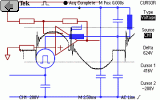Electrotechnics

# A Design of Toroidal Chokewww.e-rokodelnica.si

In the following article, a design of a toroidal choke is presented. Although there is not much left over to invent, it is still possible to make things more complex. Once I designed an air toroidal choke. Its inductance was given. So, an outer and inner diameters, height and number of turns had to be determined. These were four variables and only one inductance. Which combination of dimensions would give the optimal choke? What would be the optimal choke anyhow? To me, such choke is small so that it does not occupy much space. Besides, a winding should not have many turns because I don't have a machine to wind it. A low number of turns mean also a low resistance.

The objective of the article is to answer the question:

How would the smallest toroidal choke look like?

The reasoning in the article is valid for all types of toroidal chokes although I take a different way to design a choke with ferrite or similar core. In later case, there are only limited numbers of cores in datasheets. Next, only a few of them are available to us - mortals. So, a core is selected first. Then, number of turns that gives needed inductance is calculated. It should be checked, when it is possible to wind the choke with a wire, that would not glow. Core saturation should be checked also. And finally, I try a bigger core...## Symbols

The MKS system is going to be used in the article.

 Symbol Description L Inductance D Diameter of wire with safety margin R1 Inner radius of a core R2 Outer radius of a core h Height of a core N Number of turns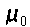Permeability of empty space (1.257 10-6Vs/Am) V Outer volume of a core l Length of wire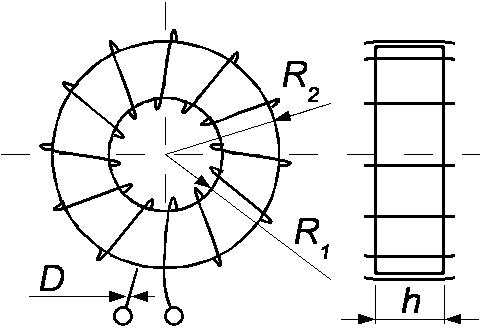## Mathematical stuff

The main parameter of a choke is its inductance. Although I often have a felling, that its price is even more important. An equation (1) which is usually used for calculation of toroidal choke is known.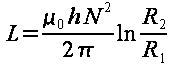(1)

There are four variables that must be set to get the wanted inductance L. These variables are inner radius R1, outer radius R2, height h and number of turns N. Because of these variables, there are many possibilities to design a choke. The question is, how to choose among them to get optimal choke. To me, the optimal choke would be a small one with low ohmic losses and with high resonant frequency.

First, only one layer of windings is going to be used. An interwinding capacitance is kept low this way. This constrain links the number of windings N with inner radius of the core R1. This relation is described by an equation (2), where a wire diameter D is introduced. This diameter is determined by a RMS of current and environment of the choke.

To be more accurate, a half of wire diameter D should be subtracted from radius in the equation (2). The error is going to be solved by using bigger diameter D as can be seen in the example later.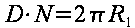(2)

Additionally, I want the choke to be small. That is why I need an equation (3) which describes a space occupied by the choke.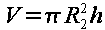(3)

The equations (1) to (3) give us an equation (4).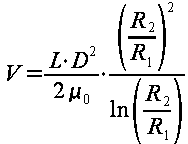(4)

This equation (4) is interesting because only a ratio of inner and outer radius is left. Which is the ratio that gives the smallest volume V? To find that out, next equation (5) must be resolved.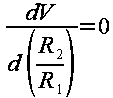(5)

And the solution is equation (6).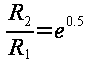(6)

The smallest toroidal choke always has the ratio between the outer radius R2 and inner radius R1 equal to 1.65. This ratio of radii is very close to the ratio named golden ratio or golden cut, which is 1.61. So, the smallest toroidal choke is also beautiful from aesthetic point of view. Nice, isn't it.

At the moment, we have four variables and three equations (1), (2) and (6). That allows us to introduce another constrain. It would be nice, when an ohmic resistance of the winding would be the smallest possible. Diameter of the wire D is given. Equation (7) describes the length of the wire l.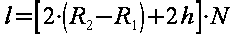(7)

In the equation (7) is made the same error as in equation (2).

An equation (8) can be assembled from equations (1), (2) and (7). When the equation (6) is added, we get equation (9).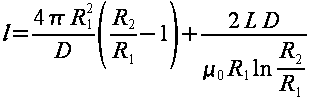(8)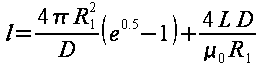(9)

We must find a radius R1 which gives the shortest length of the wire. The way to find the radius is the same as in equation (5). Again, we use a derivative.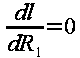(10)

The optimal inner radius R1 would be as in equation (11).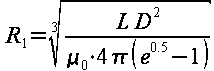(11)

The outer radius R2 is obtained from ratio in equation (6). The height of the core h and number of turns N are obtained by using equations (1) and (2). And here we have a choke.

## Example

Problem: An inductance of an air choke is 50uH. The RMS of the current is 2.5A. What are the dimensions of the smallest air choke possible?

 The diameter of the wire is determined by the RMS of current and by environment. The topic is too large to be discussed here. Nevertheless, I would advise to measure the temperature rise after the choke is made. 0.85mm Inductance is given L = 50uH A wire diameter should be increased because of the simplification in equation (2) and because of wire insulation. D = 1.1mm Equation (11) R1 = 18mm Equation (6) R2 = 30mm Equation (2) N = 103 Equation (1) h = 46mm Number of turns of wire 0.85 (in one layer) possible 122 (> N) Length of a wire by equation (7) 11.95m Actual length of a wire is a bit longer, while there is a simplification in the equation (7) 12.14m Resistance at 20°C 370mR